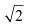• +91 9971497814
• info@interviewmaterial.com

# Chapter 14 Mathematical Reasoning Ex-14.2 Interview Questions Answers

### Related Subjects

Question 1 :
Write the negation of the following statements:
(i) Chennai is the capital of Tamil Nadu.
(ii)is not a complex number.
(iii) All triangles are not equilateral triangle.
(iv) The number 2 is greater than 7.
(v) Every natural number is an integer.

(i) Chennai is not the capital of Tamil Nadu.
(ii)is a complex number.
(iii) All triangles are equilateral triangles.
(iv) The number 2 is not greater than 7.
(v) Every natural number is not an integer.

Question 2 :
Are the following pairs of statements negations of each other?
(i) The number x is not a rational number.
The number x is not an irrational number.
(ii) The number x is a rational number.
The number x is an irrational number.

(i) The negation of the first statement is “the number x is a rational number”.
This is same as the second statement. This is because if a number is not an irrational number, then it is a rational number.
Therefore, the given statements are negations of each other.
(ii) The negation of the first statement is “the number x is not a rational number”. This means that the number x is an irrational number, which is the same as the second statement.
Therefore, the given statements are negations of each other.

Question 3 :
Find the component statements of the following compound statements and check whether they are true or false.
(i) Number 3 is prime or it is odd.
(ii) All integers are positive or negative.
(iii) 100 is divisible by 3, 11 and 5.

(i) The component statements are as follows.
p: Number 3 is prime.
q: Number 3 is odd.
Both the statements are true.
(ii) The component statements are as follows.
p: All integers are positive.
q: All integers are negative.
Both the statements are false.
(iii) The component statements are as follows.
p: 100 is divisible by 3.
q: 100 is divisible by 11.
r: 100 is divisible by 5.
Here, the statements, p and q, are false and statement r is true.

Todays Deals### Chapter 14 Mathematical Reasoning Ex-14.2 Contributorskrishan

Name:
Email:

# Latest News# 9000 interview questions in different categories